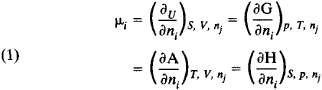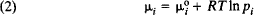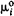# Chemical Potential

Also found in: Dictionary, Medical, Wikipedia.

## chemical potential

[′kem·i·kəl pə′ten·chəl]
(physical chemistry)
In a thermodynamic system of several constituents, the rate of change of the Gibbs function of the system with respect to the change in the number of moles of a particular constituent.
McGraw-Hill Dictionary of Scientific & Technical Terms, 6E, Copyright © 2003 by The McGraw-Hill Companies, Inc.
The following article is from The Great Soviet Encyclopedia (1979). It might be outdated or ideologically biased.

## Chemical Potential

μi, a thermodynamic variable used to describe the state of a system containing a variable number of particles. In the case of a system consisting of i components, the chemical potential is defined as the increase in internal energy U of the system upon addition of an infinitely small number of moles of the ith component expressed in relation to the quantity of this substance (with constant volume V and entropy S and a constant number of moles of each of the remaining components nj [ji]).

In general, the chemical potential can be defined as the increase in any of the remaining thermodynamic potentials of the system given various constant parameters. It can be the increase in the Gibbs free energy G at constant pressure p, temperature T, and nj; the increase in the Helmholtz free energy A at constant V, T, and nj; or the increase in the enthalpy H at constant S, p, and nj. Thus:The chemical potential depends both on the concentration of the given component and on the form and concentration of the other components (phases) of the system. Only in the simplest case, that of a mixture of ideal gases, does μi depend solely on the concentration of the component in question and the temperature:where pi is the partial pressure of component i in the mixture, R is the gas constant, andis the value of μi at pi = 1 atmosphere. For a mixture of nonideal gases, the fugacity of the component must be included in equation (2).

The chemical potential characterizes the capacity of a particular component to leave a given phase (by evaporation, dissolution, crystallization, chemical reactions). In multiphase (heterogeneous) systems, the transition of a particular component is spontaneous only when the component moves to a phase in which its chemical potential is lower. A transition of this type is accompanied by a decrease in the chemical potential of this component in the first phase and an increase in the second. As a result, the difference between the chemical potentials of the particular component in the two phases is reduced, and when equilibrium is reached the chemical potential of the component is identical in both phases. In any equilibrious heterogeneous system, the chemical potential of each component is identical in all phases.

If in various phases or at different locations in one phase the chemical potentials of some component are not identical, a spontaneous (without the expenditure of external energy) redistribution of particles will occur in the system, accompanied by equilization of the chemical potentials.

From the conditions for the thermodynamic equilibrium of systems—conditions under which chemical reactions, phase transitions, and other particle redistribution processes are possible—and from the equation describing the balance of particles, the most important thermodynamic relationships can be derived, among them the law of mass action, the Gibbs phase rule, and the fundamental laws of dilute solutions.

As a normalization constant, the chemical potential appears in the Boltzmann distribution and in the Bose-Einstein and Fermi-Dirac energy distributions for particles of an ideal gas. The chemical potential of a degenerate electron gas (Fermi gas) coincides exactly with the boundary Fermi energy.

The chemical potential, which was introduced by Gibbs, is expressed numerically in units of energy per unit of substance (joules/mole) or per unit of mass (joules/kg).

I. A. KUZNETSOV

References in periodicals archive ?
Here, C[O.sub.2] is termed as component "1" while a polymer as component "2." The chemical potential of 1 in the polymer phase is given by
where [m.sub.D] (T, [mu]) is the Debye screening mass at finite temperature and quark chemical potential :
Liu, "Holographic thermalization with a chemical potential in Gauss-Bonnet gravity," Journal ofHigh Energy Physics, vol.
The SL equation of state for the binary mixture can be used for calculating the chemical potential of C[O.sub.2] in molten polymer, [[mu].sub.1], by transforming Eq 12 into Eq 14:
where effective baryon energy is [E.sup.*.sub.[alpha]] = [([k.sup.2.sub.[alpha]] + [M.sup.*2.sub.[alpha]]).sup.1/2] and effective baryon chemical potential is [v.sub.[alpha]] = [[mu].sub.[alpha]] - [g.sub.[omega][alpha]] [[omega].sub.0] - [g.sub.[phi][alpha]] [[phi].sub.0] - [g.sub.[rho][alpha]][[tau].sub.3[alpha]] [[rho].sub.03].